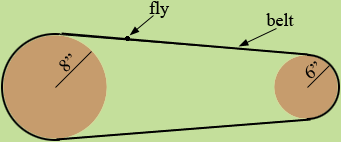SEARCH HOMEMath Central Quandaries & QueriesQuestion from Jules, a student: Can you please help me to find the answer to this problem, and please give me other formulae about Pulley. I like practicing Maths, and this question is from my mathematics book: A dead fly is stuck to a belt that passes over two pulleys 6 inches and 8 inches in radius. Assuming no slippage,how fast is the fly moving when the large pulley (8 inches) makes 21 revolutions per second ? How many revolutions per second does the small pulley make ? How long will it take the dead fly to travel 1 mile ? Thank you.Julius,The circumference of the 8 inch pulley is 2 π r = 16 π inches. The 8 inch pulley is revolving at the rate of 21 revolutions every second. Hence in one second the belt, and thus the fly, travels 21 × 16 π inches and therefore the fly is moving at 21 × 16 π inches per second. How long will it take for the fly to travel a mile?

I converted the angular velocity of the 8 inch pulley (in revolutions per second) to linear velocity of the belt (in inches per second). The belt is moving at this velocity over the 6 inch pulley also. Use this to calculate the angular velocity of the 6 inch pulley.

PennyMath Central is supported by the University of Regina and The Pacific Institute for the Mathematical Sciences.